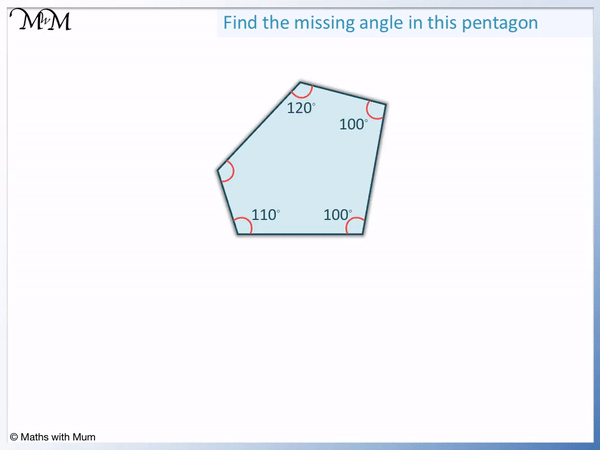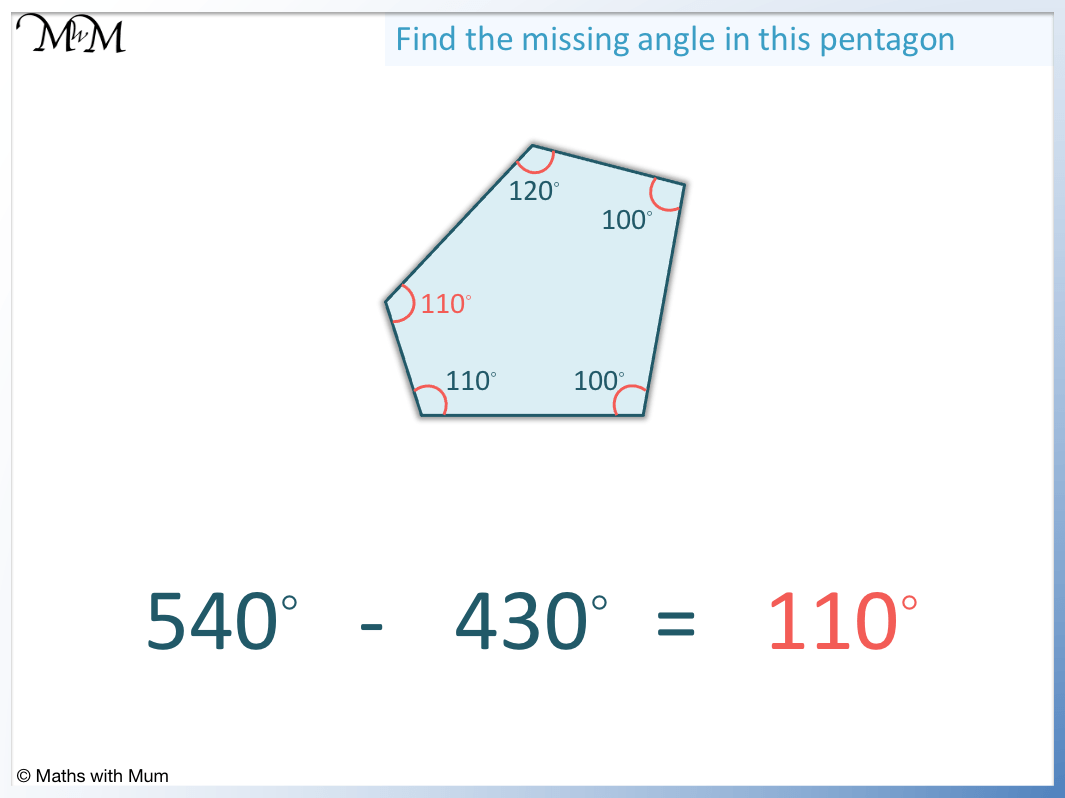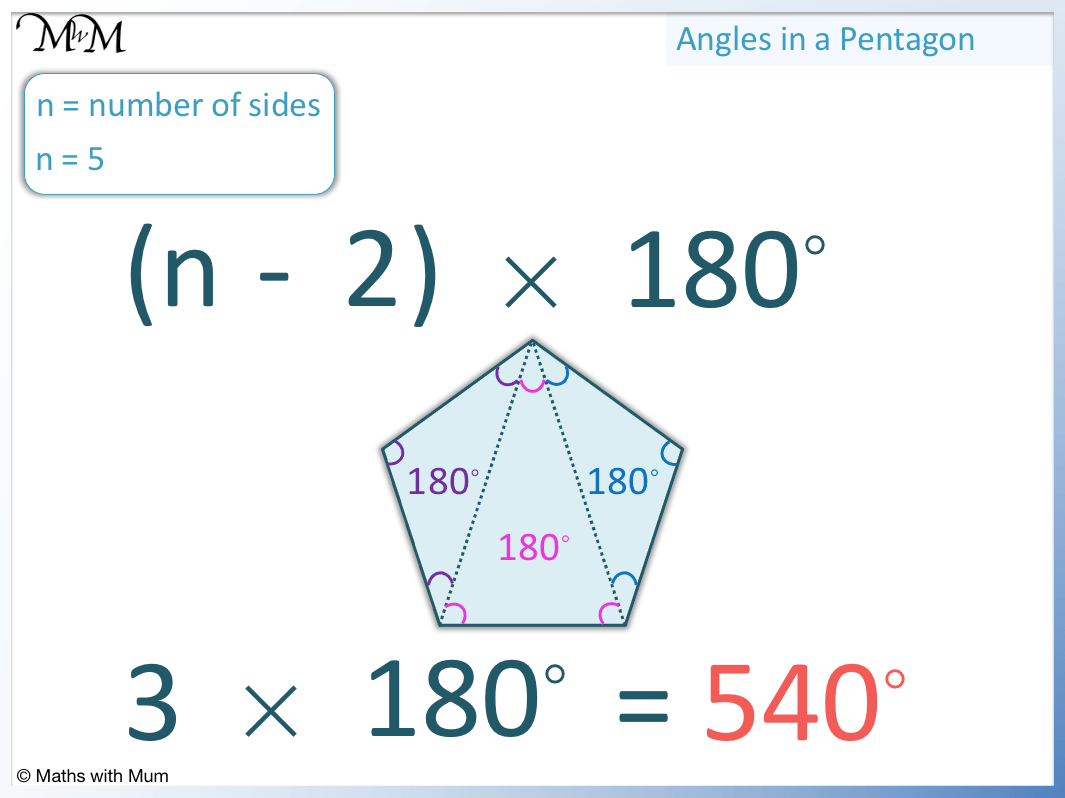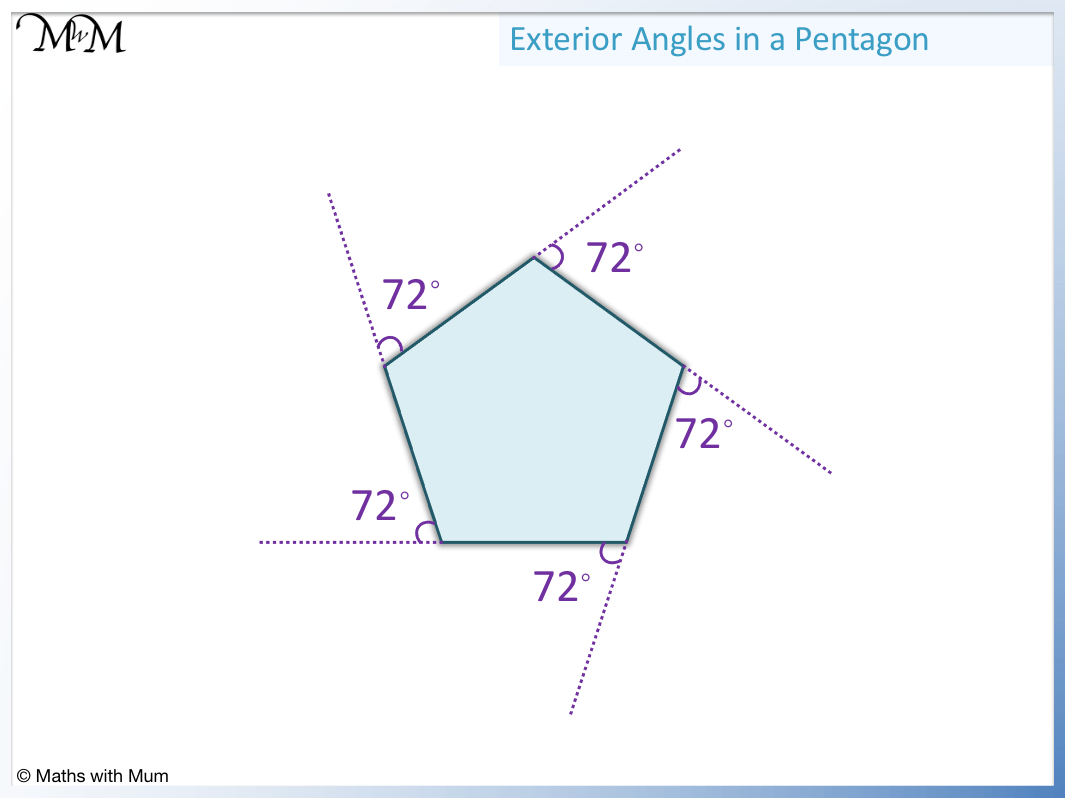# Angles in a Pentagon

Angles in a Pentagon• A pentagon is a 5-sided shape.
• Angles in pentagons always add up to 540°.
• To find a missing angle in a pentagon, add up the 4 known angles and subtract this from 540°.
• We first add the 4 known angles: 120° + 100 + 100 + 110° = 430°.
• We then subtract this from 540°: 540 – 430 = 110 and so the missing angle is 110°.
Angles in a pentagon always add up to 540°.• Angles in a pentagon always add to 540°.
• A regular pentagon is a pentagon that has 5 angles that are all the same size.
• To find the size of each angle in a regular pentagon, we simply divide 540° equally into 5 parts.
• 540° ÷ 5 = 108° and so each angle in a regular pentagon is 108°.# Angles in Pentagons

## What do Angles in a Pentagon Add Up To?

The sum of the 5 interior angles in any pentagon is always equal to 540°.

We can see that the angles in the pentagon below all add up to 540°.## How to Find a Missing Angle in a Pentagon

To find a missing angle in a pentagon, add up all of the other known angles then subtract this sum from 540°.

For example, here is a pentagon with known angles of 110°, 130°, 80° and 160°.The first step is to add the known angles together.

110 + 130 + 80 + 160 = 480°.

The second step is to subtract this total from 540°.

540° – 480° = 60° and so, the missing angle is 60°.Here is an example of finding more than one missing angle in a pentagon.

In this example, the angles in the pentagon have lines on them. This tells us that some angles are the same size.

The two angles that both have one line on them are equal to each other. Therefore angle a = 120°.We also know that angles b and c are equal to each other because they both have 2 lines on them.

To work out the size of angles b and c, we will first find the sum of the three angles above.

100° + 120 ° + 120° = 340°.

We can subtract this from 540° to get 200°.

This means that angles b and c must add together to make 200°. Since they are both the same size, we will just divide 200° by 2.

Angle b = 100° and angle c = 100°.

## Angles in a Regular Pentagon

Each interior angle in a regular pentagon is equal to 108°. This is because the sum of all 5 interior angles in any pentagon is 540°. In a regular pentagon, all five angles are of equal size and so, we divide 540° by 5 to get 108°.

Below is a regular pentagon.

If a shape is regular, this means that all of its sides are the same length and all of its angles are the same size.Angles in a pentagon add up to 540°.

Since all 5 angles are the same size, the total of 540° is shared evenly into 5 equal parts.

540° ÷ 5 = 108° and so each angle is 108°.We can check the result by adding our angles.

108° + 108° + 108° + 108° + 108° = 540°.

## Formula to Find the Angles in a Pentagon

The formula for the sum of interior angles in a polygon is (n-2) × 180°, where n is the number of sides. A pentagon has 5 sides and so, n = 5. The formula (n-2) × 180° becomes 3 × 180° = 540°. Therefore the sum of angles in a pentagon is 540°.The formula works because it tells us how many triangles can be formed inside each shape.

The number of triangles that can be drawn is equal to 2 less than the number of sides, or n – 2.

Each triangle contributes 180° to the sum of the interior angles and so, we have (n-2) × 180°.

## Why do Angles in a Pentagon Add to 540?

Angles in a pentagon add to 540° because three triangles can be made inside any pentagon by drawing lines from one corner to each of the other corners. Each triangle contains 180° and 3 × 180° = 540°.

Below is a pentagon divided into 3 triangles.

These triangles have been formed by taking one corner of the shape and drawing straight lines to each of the other corners.Each triangle contains 180°.

180° + 180° +180° = 540° and so, the sum of the three triangles is 540°.

The angles in all three triangles form the interior angles of the pentagon and so the sum of angles in a pentagon equals 540°.

## Exterior Angles of a Pentagon

Exterior angles of all pentagons add up to 360°. In a regular pentagon, each exterior angle is 72°. This is because each angle is the same size and 360° ÷ 5 = 72°.

Exterior angles of all polygons always add up to 360°.

We can see the 5 exterior angles of a regular pentagon marked below.Exterior angles add up to 360° because each polygon can be shrunk down until it forms a point. All that is left are the exterior angles. Angles around a point add to 360° and so, the exterior angles add to 360°.

This is shown below.Now try our lesson on How to Find the Area of a Parallelogram where we learn how to find the area of a parallelogram.